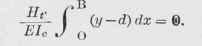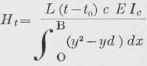The provision which should be made for temperature stresses in a concrete arch, is often a very serious matter, for the double reason that the stresses are sometimes very great, and also because the whole subject is frequently neglected. It will be shown later that the stresses due to certain assumed changes of temperature may be greater than those due to loading. There is much uncertainty regarding the actual temperature which will be assumed by a large mass of concrete. The practice which is common and proper with metal structures, is. not applicable to masonry arches.

A steel bridge, with its high thermal conductivity, will readily absorb or discharge heat; and it is usually assumed that it will readily acquire the temperature of the surrounding air. On the other hand, concrete is relatively a non-conductor. No matter what changes of temperature may take place in the outer air, the interior of the concrete will change its temperature very slowly. One test bearing on this subject was conducted by burying some electrically recording thermometers in the interior of a large mass of concrete, and recording the temperatures as they varied for a period of ten months, which included a winter season. It was found that the total variation of temperature was but a few degrees.

It is probably safe to assume that even during the coldest of winter weather the temperature of the interior of a large mass of concrete will not fall below that of the mean temperature for the month. Since the Weather Bureau records for temperate climates show that the mean temperature for a month, even during the winter months, is but little if any below freezing, it may usually be assumed that for concrete a fall of 30 degrees below the temperature of construction (say 60°) will be a sufficient allowance. A rise of temperature to 90° F. is probably much greater than would ever be found in an arch of concrete. The earth and pavement covering protect the arch from the direct action of the sun. Even in the hottest day, the space under a masonry arch seems cool, and the real temperature of the masonry probably does not exceed 70°, even if the outer air registers 95°. If we therefore calculate the stress produced by a change of temperature of 30 degrees from the temperature of construction, we are probably exceeding the real stresses produced. Even if this extreme limit should be sometimes exceeded, it simply lowers temporarily the factor of safety by a slight percentage. The following demonstration, which has been adapted from that in Church's "Mechanics" (Section 385), will be described, but without demonstrating the fundamental equations, which depend on elaborate mathematical reasoning. Assume that:

L = The length of the arch, measured in inches; t = Temperature, in degrees, Fahrenheit; therefore t - t0 represents the change of temperature expressed in degrees, Fahrenheit; c = Coefficient of expansion for concrete, which is here assumed to be 0000065;

Ht = Imaginary horizontal forces which would produce the same stress on the arch as that produced by any assumed change of temperature; d = Height of the line of action of Ht above the abutment level.

The other symbols are as previously used. In Fig. 233, the heavy full line between O and B represents the arch rib in its normal and unstrained condition. If it were free to expand, it would assume some such form as indicated by the dotted line O'C'B'; but since the arch is fixed at the abutments, the arch rib is forced to preserve the same distance between the abutments, and the tangents to the rib at the abutments remain fixed in direction. The rib therefore is forced to the form 0 C" B. Of course the relative distortion is enormously exaggerated. These two requirements furnish the data for the equations given below. If the arch rib were assumed to be extended by the addition of the imaginary arms as shown, and the two equal and opposite forces (Ht) were acting as indicated, these forces would hold the arch rib rigid against the tendency to expand or to change its direction at the abutments. Considered as an example of the general case of an arch rib acted on by forces, we may consider that the arch rib is acted on only by these two equal and opposite forces Ht. Their line of action is the vm of the problem, as previously explained; and d is therefore the distance from the abutment up to this line vm. The equation which is based on the fact that the span of the arch is not changed by the temperature, or that it does not expand from OB to O'B', is as follows:Fig. 233. Temperature Stresses in Arch Rib.The fact that there was no change in direction of the tangents, gives rise to the equation:As before, we must allow for the variable moment of inertia, 7;.but since I = nlc, and ds = ndx, then ds/I = dx/Ic, and Equation 61 becomes:But since Ht, E, and Ic are all constants, we may drop them in this case, and write the equation:Since d is a constant, this equation virtually means that the summation of all the y's between B and O equals d times the number of sections. But the summation of the y's, as shown in the tabular form in Article 434, is 212.90 feet, or 2,544.8 inches. Dividing this by 20, we have 127.24 inches, which is the value of d, or the height of vm above the abutment, and is the distance above the abutment of the line of action of the pair of imaginary forces Ht which will produce the same stress in the arch as the assumed change in temperature. Equation 60 may be transposed so as to solve for Ht and we may write (substituting dx/1 for ds/1):For practical use, we transform the denominator of the above expression into the summation of y2 - yd for each point, times the constant distance between these points (= 3.07 feet, or 36.84 inches). The various values of y are obtained by taking the figures in the first column of the tabular form in Article 434, and multiplying each by 12 to reduce to inches. Squaring the several values of \2y for half of the span, and adding the squares, we may multiply the sum by 2, and obtain 381,328 as the sum of the squares; but the sum of all the y's, times 12, equals 2,544.8, and we may multiply this by 122.74, and obtain 313,576 as the summation of yd. Subtracting this from the summation of y2, we have left 67,752, which, multiplied by the value of dx in inches (36.84), gives 2,495,9S4, which is the denominator of the above fraction. Computing Ic, the moment of inertia of the arch rib at the center, in a similar manner to the computation in Article 437, we find a value of 6,529 biquadratic inches.

Substituting all the known values in the above equation, we have:

720 x 30 x .0000005 x 2.400,000 x 6,529

H t= -------------------------------------------------- = 881 2,495,984

(y - d) for center = 183 - 127.2 = 55.8 (y - d) for abutments = 0 - 127.2 = - 127.2.

The moment produced by the assumed change of temperature of 30 degrees, is therefore as follows:

At the center, 881 X 55.8 = 49,160 inch-pounds.

At the abutments, 881 X (- 127.2) = - 112,003 inch-pounds.

It was computed above, that a moment of 149,490 inch-pounds at the abutment would produce a unit-stress in the concrete of 151 pounds per square inch; therefore a unit-stress produced in the abutment by a moment of 112,063 pounds would be -112,063 X 151

149,490